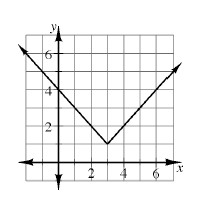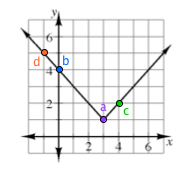### Home > AC > Chapter 12 > Lesson 12.1.3 > Problem12-26

12-26.

Examine the graph of $f ( x ) = | x - 3 | + 1$ at right. Use the graph to find the values listed below.

1. $f(3)$

1. $f(0)$

1. $f(4)$

1. $f(− 1)$The number in parentheses is the x-value that corresponds with a point on the function. Find that point. Substitute the given x into the equation.